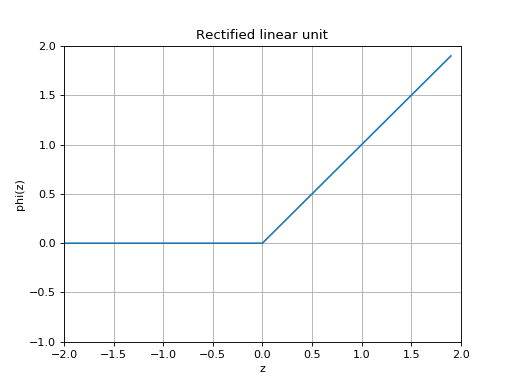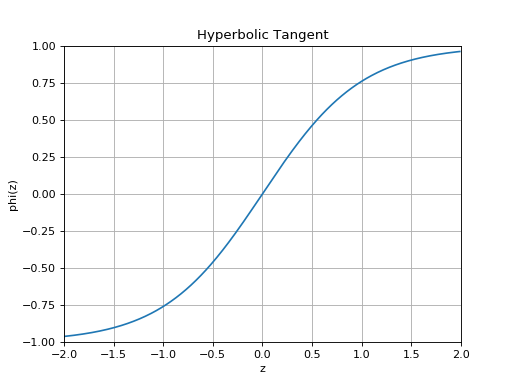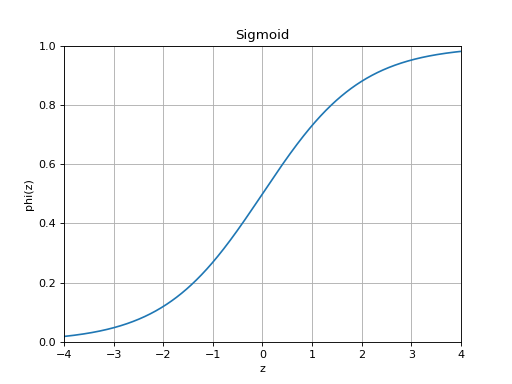# Activation Functions¶

Neural networks rely on a nonlinear transformation to learn nonlinear relationships in data. These nonlinear transformations are typically fixed functions that are applied after a linear transformation of the data. The linear transformation uses learned weights, while the nonlinear function is fixed in that there are no learned parameters. In most cases, these nonlinear functions can be thought of as activation functions that indicate the state of a unit within a layer of a neural network, given some data.

class slugnet.activation.ReLU[source]

Bases: slugnet.activation.Activation

The common rectified linean unit, or ReLU activation funtion.

A rectified linear unit implements the nonlinear function.class slugnet.activation.Tanh[source]

Bases: slugnet.activation.Activation

The hyperbolic tangent activation function.

A hyperbolic tangent activation function implements the nonlinearity given by, which is equivalent to.class slugnet.activation.Sigmoid[source]

Bases: slugnet.activation.Activation

Represent a probability distribution over two classes.

The sigmoid function is given by.class slugnet.activation.Softmax[source]

Bases: slugnet.activation.Activation

Represent a probability distribution overclasses.

The softmax activation function is given bywhereis the number of classes. We can see that softmax is a generalization of the sigmoid function toclasses. Below, we derive the sigmoid function using softmax with two classes.We substitutebecause we only need one variable to represent the probability distribution over two classes. This leaves us with the definition of the sigmoid function.